# Steenrod algebra

(diff) ← Older revision | Latest revision (diff) | Newer revision → (diff)

The graded algebra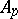over the field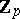of all stable cohomology operations (cf. Cohomology operation) modulo. For any space (spectrum of spaces), the group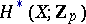is a module over the Steenrod algebra.

The Steenrod algebra is multiplicatively generated by the Steenrod operations (cf. Steenrod operation). Thus, the Steenrod algebra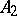is a graded associative algebra, multiplicatively generated by the symbolswith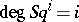, which satisfy the Adem relation:, so that an additive basis (over) of the Steenrod algebraconsists of the operations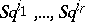,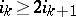(the so-called Cartan–Serre basis). Similar results are true forwith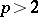. Furthermore,where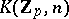is an Eilenberg–MacLane space. The multiplication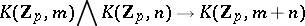induces the diagonalin, which is a homomorphism of algebras, and, consequently, turnsinto a Hopf algebra.

How to Cite This Entry:
Steenrod algebra. Encyclopedia of Mathematics. URL: http://encyclopediaofmath.org/index.php?title=Steenrod_algebra&oldid=48824
This article was adapted from an original article by Yu.B. Rudyak (originator), which appeared in Encyclopedia of Mathematics - ISBN 1402006098. See original article